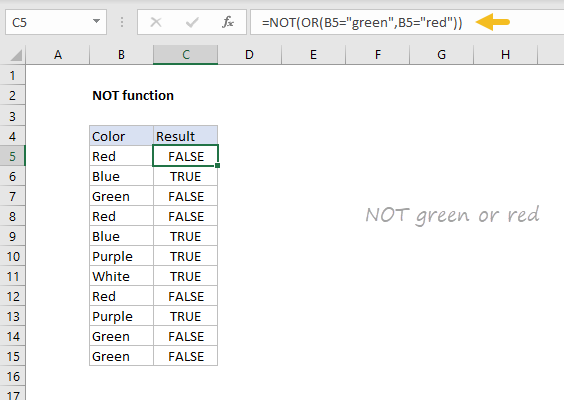Purpose
Reverse arguments or results

Return value
A reversed logical value

Arguments
logical - A value or logical expression that can be evaluated as TRUE or FALSE.

Syntax
=NOT(logical)

Example #1 - not green or red
In the example shown, the formula in C5, copied down, is:
=NOT(OR(B5="green",B5="red"))
The literal translation of this formula is "NOT green or red". At each row, the formula returns TRUE if the color in column B is not green or red, and FALSE if the color is green or red.

Example #2 - Not blank
A common use case for the NOT function is to reverse the behavior of another function. For example, If cell A1 is blank (empty), the ISBLANK function will return TRUE:
=ISBLANK(A1)  // TRUE if A1 is empty

To reverse this behavior, wrap the NOT function around the ISBLANK function:
=NOT(ISBLANK(A1))  // TRUE if A1 is NOT empty

By adding NOT the output from ISBLANK is reversed. This formula will return TRUE when A1 is not empty and FALSE when A1 is empty. You might use this kind of test to only run a calculation if there is a value in A1:
=IF(NOT(ISBLANK(A1)),B1/A1,"")